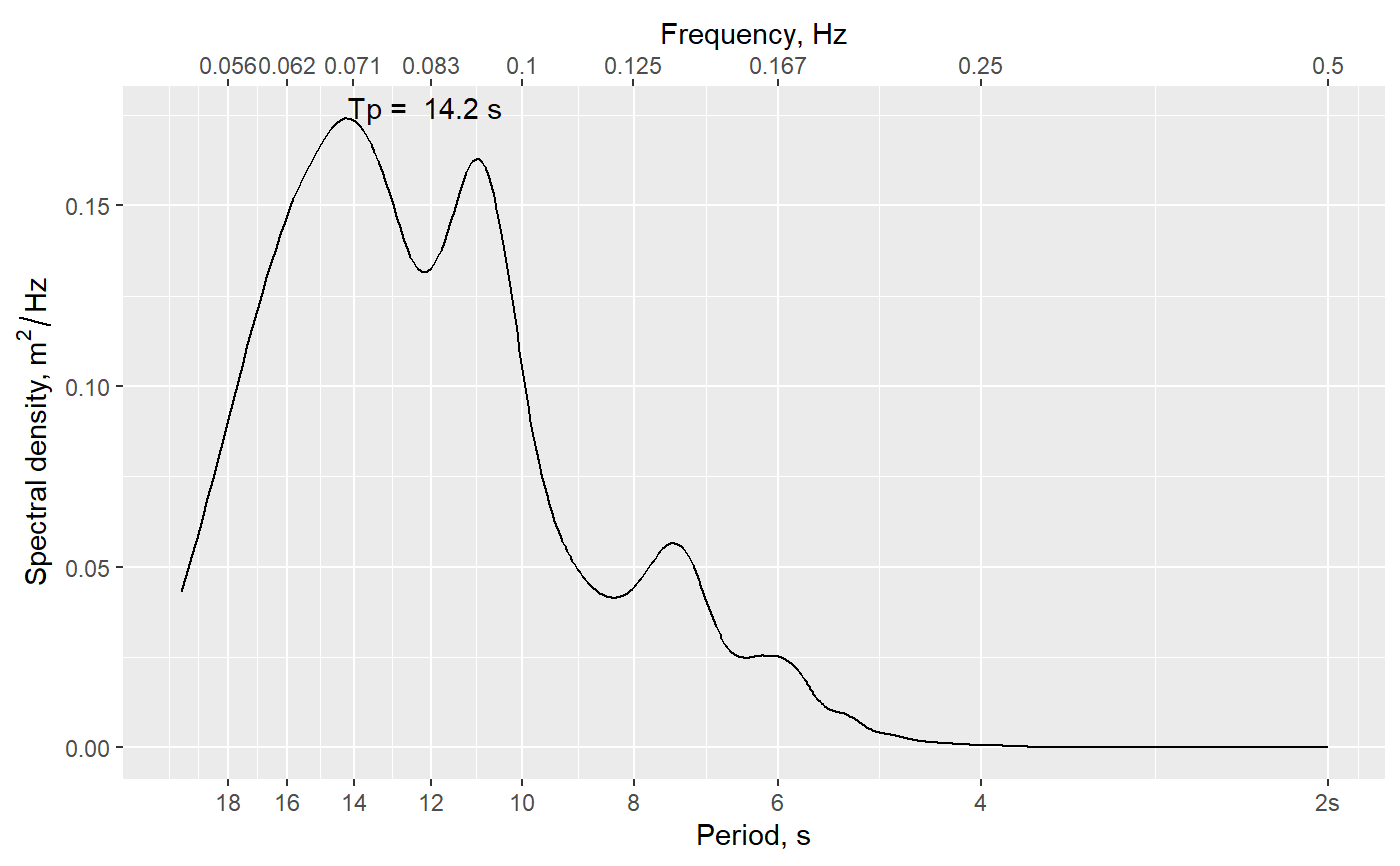Calculate ocean wave parameters using spectral analysis methods

waveStatsSP(data, Fs, method = c("welchPSD", "spec.pgram"),
plot = FALSE, kernel = NULL, segments = NULL, ...)

## Arguments

data A vector of surface heights that constitute a time series of observations. Typical units = meters. Sampling frequency of the surface heights data. Units = Hz, i.e. samples per second. A character string indicating which spectral analysis method should be used. Choose one of welchPSD (default) or spec.pgram. A logical value denoting whether to plot the spectrum. Defaults to FALSE. An object of class tskernel that defines a smoother for use with spec.pgram method. If value is NULL, a default Daniell kernel with widths (9,9,9) is used. Numeric value indicating the number of windowing segments to use with welchPSD method. Additional arguments to be passed to spectral analysis functions, such as the windowfun option for welchPSD.

## Value

List of wave parameters based on spectral methods.

• h Average water depth. Same units as input surface heights (typically meters).

• Hm0 Significant wave height based on spectral moment 0. Same units as input surface heights (typically meters). This is approximately equal to the average of the highest 1/3 of the waves.

• Tp Peak period, calculated as the frequency with maximum power in the power spectrum. Units of seconds.

• m0 Estimated variance of time series (moment 0).

• T_0_1 Average period calculated as $$m0/m1$$, units seconds. Follows National Data Buoy Center's method for average period (APD).

• T_0_2 Average period calculated as $$(m0/m2)^0.5$$, units seconds. Follows Scripps Institution of Oceanography's method for calculating average period (APD) for their buoys.

• EPS2 Spectral width parameter.

• EPS4 Spectral width parameter.

## Details

Carries out spectral analysis of ocean wave height time series to estimate common wave height statistics, including peak period, average period, and significant wave height.

Original MATLAB function by Urs Neumeier: http://neumeier.perso.ch/matlab/waves.html, based on code developed by Travis Mason, Magali Lecouturier and Urs Neumeier.

waveStatsZC for wave statistics determined using a zero-crossing algorithm.

## Examples

data(wavedata)
waveStatsSP(wavedata$swDepth.m, Fs = 4, method = 'spec.pgram', plot = TRUE)#>$h
#>  10.45138
#>
#> $Hm0 #>  0.4146835 #> #>$Tp
#>  14.17323
#>
#> $m0 #>  0.01074765 #> #>$T_0_1
#>  10.47688
#>
#> $T_0_2 #>  9.782931 #> #>$EPS2
#>  0.3832773
#>
#> \$EPS4
#>  0.6402274
#>